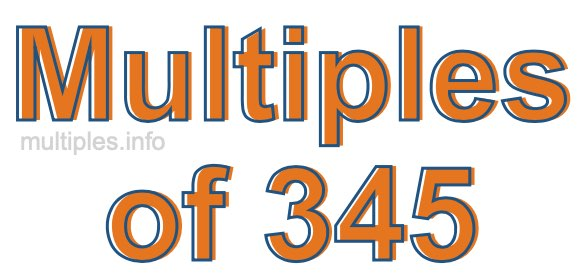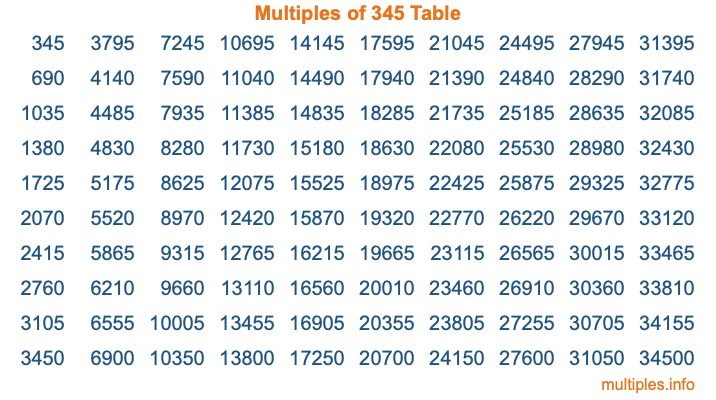Multiples of 345Welcome to the Multiples of 345 page. Here we will first teach you everything you will ever need to know about the multiples of 345, and then give you a study guide summary of everything we taught you to make sure you remember it all. Use this page to look up facts and learn information about the multiples of 345. This page will make you a multiples of three hundred forty-five expert!

Definition of Multiples of 345
Multiples of 345 are all the numbers that when divided by 345 equal an integer. Each of the multiples of 345 are called a multiple. A multiple of 345 is created by multiplying 345 by an integer.

Therefore, to create a list of multiples of 345, you start with 1 multiplied by 345, then 2 multiplied by 345, then 3 multiplied by 345, and so on for as long as you want. Thus, the list of the first five multiples of 345 is 345, 690, 1035, 1380, and 1725. To see a larger list of multiples of 345, see the printable image of Multiples of 345 further down on this page. We also have a category where you can choose any nth multiple of 345.

Multiples of 345 Checker
The Multiples of 345 Checker below checks to see if any number of your choice is a multiple of 345. In other words, it checks to see if there is any number (integer) that when multiplied by 345 will equal your number. To do that, we divide your number by 345. If the the quotient is an integer, then your number is a multiple of 345.

Is  a multiple of 345?

Least Common Multiple of 345 and ...
A Least Common Multiple (LCM) is the lowest multiple that two or more numbers have in common. This is also called the smallest common multiple or lowest common multiple and is useful to know when you are adding our subtracting fractions. Enter one or more numbers below (345 is already entered) to find the LCM.

Check out our LCM Calculator if you need more details about the Least Common Multiple or if you need the LCM for different numbers for adding and subtraction fractions.

nth Multiple of 345
As we stated above, 345 is the first multiple of 345, 690 is the second multiple of 345, 1035 is the third multiple of 345, and so on. Enter a number below to find the nth multiple of 345.

th multiple of 345

Multiples of 345 vs Factors of 345
345 is a multiple of 345 and a factor of 345, but that is where the similarities end. All postive multiples of 345 are 345 or greater than 345. All positive factors of 345 are 345 or less than 345.

Below is the beginning list of multiples of 345 and the factors of 345 so you can compare:

Multiples of 345: 345, 690, 1035, 1380, 1725, etc.

Factors of 345: 1, 3, 5, 15, 23, 69, 115, 345

As you can see, the multiples of 345 are all the numbers that you can divide by 345 to get a whole number. The factors of 345, on the other hand, are all the whole numbers that you can multiply by another whole number to get 345.

It's also interesting to note that if a number (x) is a factor of 345, then 345 will also be a multiple of that number (x).

Multiples of 345 vs Divisors of 345
The divisors of 345 are all the integers that 345 can be divided by evenly. Below is a list of the divisors of 345.

Divisors of 345: 1, 3, 5, 15, 23, 69, 115, 345

The interesting thing to note here is that if you take any multiple of 345 and divide it by a divisor of 345, you will see that the quotient is an integer.

Multiples of 345 Table
Below is an image of the first 100 multiples of 345 in a table. The table is in chronological order, column by column. The first column has the first ten multiples of 345, the second column has the next ten multiples of 345, and so on.The Multiples of 345 Table is also referred to as the 345 Times Table or Times Table of 345. You are welcome to print out our table for your studies.

Negative Multiples of 345
Although not often discussed or needed in math, it is worth mentioning that you can make a list of negative multiples of 345 by multiplying 345 by -1, then by -2, then by -3, and so on, to get the following list of negative multiples of 345:

-345, -690, -1035, -1380, -1725, etc.

Multiples of 345 Summary
Below is a summary of important Multiples of 345 facts that we have discussed on this page. To retain the knowledge on this page, we recommend that you read through the summary and explain to yourself or a study partner why they hold true.

There are an infinite number of multiples of 345.

A multiple of 345 divided by 345 will equal a whole number.

345 divided by a factor of 345 equals a divisor of 345.

The nth multiple of 345 is n times 345.

The largest factor of 345 is equal to the first positive multiple of 345.

345 is a multiple of every factor of 345.

345 is a multiple of 345.

A multiple of 345 divided by a divisor of 345 equals an integer.

345 divided by a divisor of 345 equals a factor of 345.

Any integer times 345 will equal a multiple of 345.

Multiples of a Number
Here you can get the multiples of another number, all with the same attention to detail as we did for multiples of 345 on this page.

Multiples of
Multiples of 346
Did you find our page about multiples of three hundred forty-five educational? Do you want more knowledge? Check out the multiples of the next number on our list!